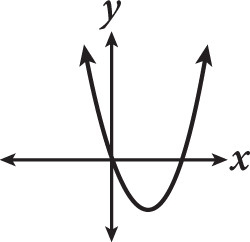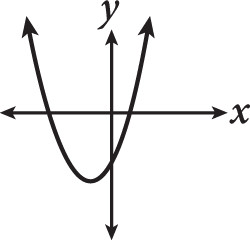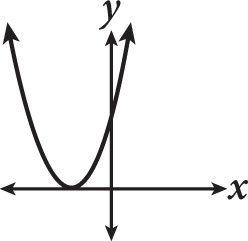# SAT Math Multiple Choice Question 424: Answer and Explanation

### Test Information

Question: 424

4. If a = 0 and b < 0, then which of the following could be the graph of f(x) = (x – a)(x – b)?

• A.• B.• C.• D.Explanation:

A

Difficulty: Medium